If you have a related question, please click the "Ask a related question" button in the top right corner. The newly created question will be automatically linked to this question.

# TPS55010: TPS55010

Part Number: TPS55010

hello,
it is not new question, but supplement to my related question (unfortunatelly my previous qestion has been locked).
I have design small transformer for 2x5V output voltage.
I have used core with nominal AL=1200 [nH/Ts2] (core without air gap).
Primary winding consist of 2 turn (8 paralel wires - litz wire) and each secondary consist 5 turn (2 paralel wires - litz wire).
Primary inductance is 5,3uH and primary resistance is 53mOHM.
Secondary inducatnce is 28,9uH and 29,2uH and secondary resistance is 85mOHM and 85mOHM.

Converter with this transformer work wery well.

Primary voltage: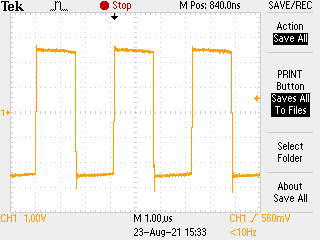Secondary voltage: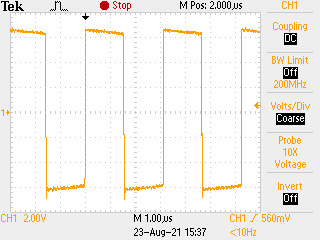• Hi,

Thank you for sharing the results.

Do you have any questions for us?

Regards,

Febin

• No, thanks for replay.

• Hello Wojciech,

First, I assume that you use the transformer calculation shown in section 9.4.2 of the datasheet. Is it correct?

In the Flybuck converter, the energy is stored on the center leg of the transformer. Hence, an air gap is typically necessary to avoid transformer saturation. You can use the formula below to calculate the max flux density (Bmax) to determine if the transformer will not saturate at max output power (Bmax < Bsat). Bmax specs are dependent on the core type.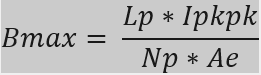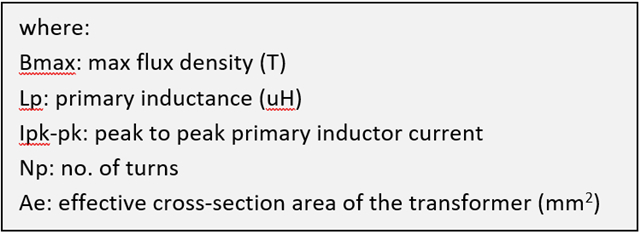Best regards,

Excel

• hello,
not exactly, there is no point 9.4.2 in my datasheet.
I have calculate Lpri(max) from 14 equation in 9.2.4 point.
Next, I have take from ferrite core datasheet max flux = 300mT, Effective area Ae=10,7mm2.
I take frequency about 350kHz (dt=~1,4us) and Upri=0,5*Uin (Upri=2,5V).
From equation Npri=((Uin-Upri)*dt)/(dB*Ae) i have calculated number of primary turns, necessery to avoid core saturation.
I have rounded number of turns up, then I calculate number of secondary turns. I obtain new value of primary voltage. And Its all :-).

• Hello Wojciech,

I made a typo error, and it should be 9.2.4. It looks like the transformer is designed properly. Hence, an air gap might not be necessary.

By the way, you can also counter-check if there is transformer saturation by measuring the current waveform across the primary winding at full load and overload/short circuit condition. Please refer to the figure below.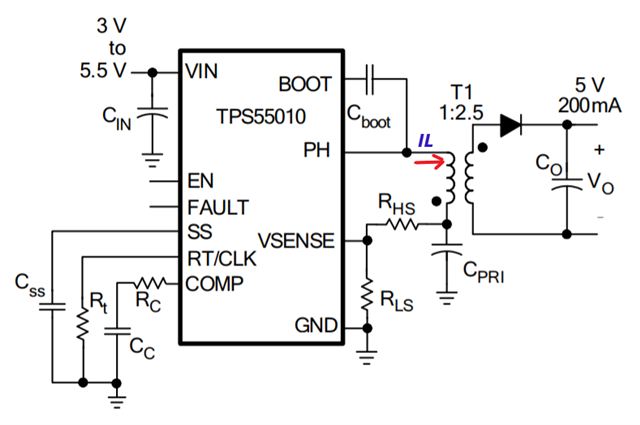Transformer waveform in saturationBest regards,

Excel

• Hi Wojciech,

If you do not have further questions, I will go ahead and close this thread. Please hit Resolved if you agree.

Regards,

Febin